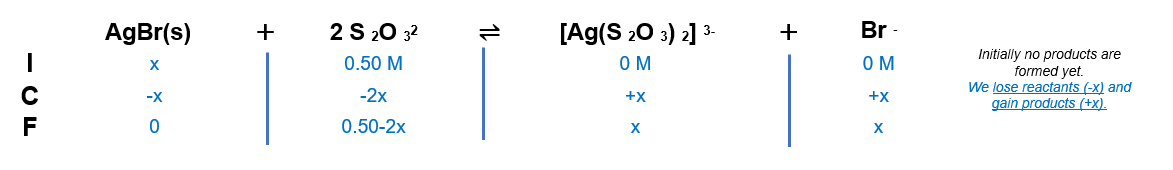# Problem: Solutions of sodium thiosulfate are used to dissolve unexposed AgBr (K sp = 5.0 X 10 -13) in the developing process for blackand- white film. What mass of AgBr can dissolve in 1.00 L of 0.500 M Na2S2O3? Ag+ reacts with S2O32- to form a complex ion:Ag+(aq) + 2S2O32-(aq) ⇌ Ag(S2O3)23-(aq)             K = 2.9 X 10 13

###### FREE Expert Solution

Eqn 1 : AgBr(s)  ⇌ Ag+(aq)   +      Br -(aq)     ;      Ksp =5.0 x 10 -13

Eqn 2 : Ag+(aq)  + 2 S2O32-(aq) ⇌  [Ag(S2O 3)2] 3-(aq)   ;      K f   = 2.9 x 10 13

_____________________________________

AgBr(s)   +   2 S2O 32-(aq)   ⇌  [Ag(S 2O 3) 2] 3- (aq)  +  Br -(aq)

We added the equations and it became exactly the overall equation.

This means we can simply multiply the constants to get the overall constant.

Keq = 14.5

'Make ICF chart:Take note that since the solution is only 1 L, moles and molarities can be interchangeable.

x = 0.2210 M

89% (79 ratings)###### Problem Details

Solutions of sodium thiosulfate are used to dissolve unexposed AgBr (K sp = 5.0 X 10 -13) in the developing process for blackand- white film. What mass of AgBr can dissolve in 1.00 L of 0.500 M Na2S2O3? Ag+ reacts with S2O32- to form a complex ion:

Ag+(aq) + 2S2O32-(aq) ⇌ Ag(S2O3)23-(aq)

K = 2.9 X 10 13